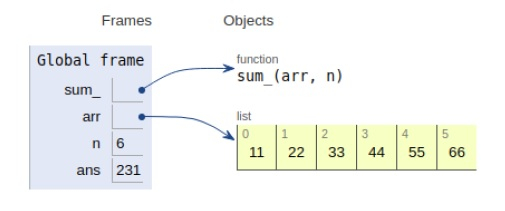# Find the sum of array in Python Program

PythonServer Side ProgrammingProgramming

Problem statement − We are given an array we need to compute the sum of the array.

The brute-force approach to traverse the whole array and element at each index to obtain the sum is discussed below at each index to obtain the sum is discussed below

## Example

Live Demo

# sum function
def sum_(arr,n):
# using built-in function
return(sum(arr))
# main
arr = [11,22,33,44,55,66]
# length
n = len(arr)
ans = sum_(arr,n)
# display sum
print("Sum of the array is ",ans)

## Output

Sum of the array is 231All the variables are declared in the local scope and their references are seen in the figure above.

## Conclusion

In this article, we have learned how to print the sum of array